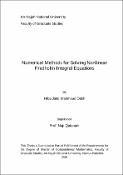## Numerical Methods for Solving Nonlinear Fredholm Integral Equations2019-11-14
Odeh, Hiba Jalal
##### Publisher
An-Najah National University
##### Abstract
In this thesis we focus on the numerical treatment of nonlinear Fredholm integral equation of the second kind due to their enormous importance in many applications in various fields. After addressing the basic concepts of nonlinear Fredholm integral equation of the second kind, we focus on the numerical treatment of this equation. This will be accomplished by implementing two numerical methods, namely, Haar Wavelet method and Homotopy Analysis method (HAM). The mathematical framework of these numerical methods will be presented. These numerical methods will be illustrated by solving some numerical examples with known exact solutions. Numerical results show clearly that the Homotopy analysis method is more effective in solving nonlinear Fredholm integral equations in comparison with its counter parts.
##### Keywords
Numerical Methods , Solving Nonlinear Fredholm Integral Equations